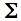t-Test for Independent or Correlated Samples

The logic and computational details of two-sample t-tests are described in Chapters 9-12 of the online text Concepts & Applications of Inferential Statistics. For the independent-samples t-test, this unit will perform both the "usual" t-test, which assumes that the two samples have equal variances, and the alternative t-test, which assumes that the two samples have unequal variances. (A good formulaic summary of the unequal-variances t-test can be found on the StatsDirect web site. A more thorough account appears in the online journal Behavioral Ecology.)

 Setup Procedure Data Entry Sample A Sample B Please be sure to perform the Data Check procedure.
 Data Summary A B Total n -X -X2 SS mean
ResultsQ
 Meana—Meanb t df
 P one-tailed two-tailed

 For independent samples, these results pertain to the "usual" t-test,which assumes that the two samples have equal variances.
F-Test for the Significance of the Difference
between the Variances of the Two SamplesQ

 df1 df2 F P [Applicable only to independent samples.] P>.05 indicates no significant difference detected between the variances of the two samples.
t-Test Assuming Unequal Sample Variances
[Applicable only to independent samples.]Q

 Meana—Meanb t df
 P one-tailed two-tailed

For purposes of significance tests and calculation of confidence intervals, values of df associated with the unequal-variance condition are rounded to the nearest integer.
 Observed Confidence Intervals 0.95 0.99 Meana ± ± Meanb ± ± Meana−Meanb [Assuming equalsample variances.] ± ± Meana−Meanb [Assuming unequalsample variances.] ± ±

 Home Click this link only if you did not arrive here via the VassarStats main page.